JMSLTM Numerical Library 7.2.0
com.imsl.datamining

## Class KohonenSOMTrainer

• All Implemented Interfaces:
Serializable

```public abstract class KohonenSOMTrainer
extends Object
implements Serializable```
Trains a Kohonen network.

`KohonenSOMTrainer` is an abstract class with 2 abstract methods, `getNeighborhoodValue` and `getLearningCoefficient`. Therefore, a subclass of `KohonenSOMTrainer` needs to implement the `getNeighborhoodValue` and `getLearningCoefficient` methods.

KohonenSOM Example, Serialized Form
• ### Constructor Summary

Constructors
Constructor and Description
`KohonenSOMTrainer()`
• ### Method Summary

Methods
Modifier and Type Method and Description
`int` `getIterations()`
Returns the number of iterations used for training.
`abstract double` `getLearningCoefficient(int t)`
Returns the learning coefficient.
`abstract double` ```getNeighborhoodValue(int t, double d)```
Returns the neighborhood function value.
`int` `getNumberOfThreads()`
Returns the number of `java.lang.Thread` instances used for parallel processing.
`void` `setIterations(int iterations)`
Sets the number of iterations to be used for training.
`void` `setNumberOfThreads(int numberOfThreads)`
Sets the number of `java.lang.Thread` instances to be used for parallel processing.
`void` ```train(KohonenSOM kohonen, double[][] data)```
Trains a Kohonen network.
• ### Methods inherited from class java.lang.Object

`clone, equals, finalize, getClass, hashCode, notify, notifyAll, toString, wait, wait, wait`
• ### Constructor Detail

• #### KohonenSOMTrainer

`public KohonenSOMTrainer()`
• ### Method Detail

• #### getIterations

`public int getIterations()`
Returns the number of iterations used for training.
Returns:
An `int` scalar containing the number of iterations used for training.
• #### getLearningCoefficient

`public abstract double getLearningCoefficient(int t)`
Returns the learning coefficient. The monotonically decreasing learning coefficient function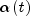is a scalar factor that defines the size of the update correction. The value ofdecreases with the step index `t`. Typical forms are linear, power, and inverse time/step. For example:

power: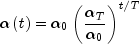where t=`t`, T=the number of iterations used for training,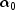= initial learning coefficient,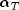= final learning coefficient

inverse time: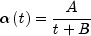where A and B are user determined constants

Parameters:
`t` - An `int` scalar containing the current iteration of the training.
Returns:
A `double` scalar containing the computed learning coefficient.
• #### getNeighborhoodValue

```public abstract double getNeighborhoodValue(int t,
double d)```
Returns the neighborhood function value. In the simplest form, the neighborhood function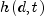is 1 for all nodes closest to the BMU and 0 for others, but a Gaussian function is also commonly used. For example: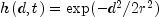where r represents the neighborhood radius at index t

Parameters:
`t` - An `int` scalar containing the current iteration of the training.
`d` - A `double` scalar containing the lattice distance between the best matching node and the current node.
Returns:
A `double` scalar containing the computed neighborhood function value.

`public int getNumberOfThreads()`
Returns the number of `java.lang.Thread` instances used for parallel processing.
Returns:
an `int` containing the number of `java.lang.Thread` instances used for parallel processing.
• #### setIterations

`public void setIterations(int iterations)`
Sets the number of iterations to be used for training.
Parameters:
`iterations` - An `int` scalar containing the number of iterations to be used for training. `iterations` must be greater than zero.

Default: `iterations` = 100.

`public void setNumberOfThreads(int numberOfThreads)`
Sets the number of `java.lang.Thread` instances to be used for parallel processing.
Parameters:
`numberOfThreads` - an `int` specifying the number of `java.lang.Thread` instances to be used for parallel processing.

Default: `numberOfThreads` = 1.

• #### train

```public final void train(KohonenSOM kohonen,
double[][] data)```
Trains a Kohonen network.
Parameters:
`kohonen` - A `KohonenSOM` object to be trained.
`data` - A `double` matrix containing the data to be used for training the Kohonen network. `data[i].length` must be equal to `dim`, the number of weights for each node, in `kohonen`.
JMSLTM Numerical Library 7.2.0